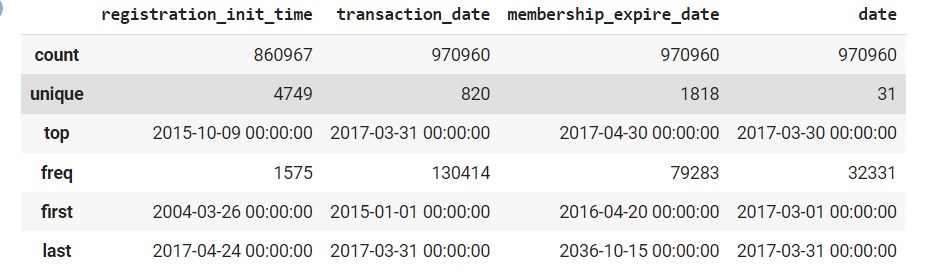top of page

# Importing, Cleaning and Visualizing Data in Python

## Introduction :

Today we are going to talk about the importance of importing ,cleaning and visualize data using a great example from Kaggle this example called customer churn prediction the aim goal of this project to predict if the customer will leave or not and try to get some action if you know that the customer will leave.

## Importing Data :

Today we will learn how to import data from Kaggle using API and download data on Google Colab notebook and the steps to do it.

1- Create Kaggle account.

3- Press to Create API token to get your own credential.

4- This file is Kaggle.json

from google.colab import files uploaded = files.upload() for fn in uploaded.keys(): print('User uploaded file "{name}" with length {length} bytes'.format( name=fn, length=len(uploaded[fn]))) # Then move kaggle.json into the folder where the API expects to find it. !mkdir -p ~/.kaggle/ && mv kaggle.json ~/.kaggle/ && chmod 600 ~/.kaggle/kaggle.json

6- Once you upload Kaggle.json now you can use Kaggle API to download data for our data we will use the following API.

!7z e members_v3.csv.7z !7z e sample_submission_v2.csv.7z !7z e train_v2.csv.7z !7z e transactions_v2.csv.7z !7z e user_logs_v2.csv.7z

8- Importing the essential libraries.

import pandas as pd import numpy as np import seaborn as sns import matplotlib.pyplot as plt

## EDA and Clean Data :

Now we have multiple tables and we need to merge them together

train = pd.merge(train,members,on='msno',how='left') test = pd.merge(test,members,on='msno',how='left') train = pd.merge(train,transactions,how='left',on='msno',left_index=True, right_index=True) test = pd.merge(test,transactions,how='left',on='msno',left_index=True, right_index=True,) train = pd.merge(train,user_log,how='left',on='msno',left_index=True, right_index=True) test = pd.merge(test,user_log,how='left',on='msno',left_index=True, right_index=True) del members,transactions,user_log print('Number of rows & columns',train.shape) print('Number of rows & columns',test.shape)

Get some summary statistics

train[['registration_init_time' ,'transaction_date','membership_expire_date','date']].describe()Now it`s time to check if there is a missing values

train.isnull().sum()Once we know that we have missing values it`s time to handle it we create a function to handle missing values

col = [ 'city', 'bd', 'gender', 'registered_via'] def missing(df,columns): col = columns for i in col: df[i].fillna(df[i].mode(),inplace=True) missing(train,col) missing(test,col)

Once we clean our data it`s time to visual our data to share our findings

## Visualizing Data :

We want to answer this question The subscription within 30 days of expiration is True/False using a perfect graph

plt.figure(figsize=(8,6)) sns.set_style('ticks') sns.countplot(train['is_churn'],palette='summer') plt.xlabel('The subscription within 30 days of expiration is True/False')As we see in the graph above the number of the subscription is true is small but we need to have save these customers

Now we will have some univariate analysis to see the count of gender and city,...etc

print(train['city'].unique()) fig,ax = plt.subplots(2,2,figsize=(16,8)) ax1,ax2,ax3,ax4 = ax.flatten() sns.set(style="ticks") sns.countplot(train['city'],palette='summer',ax=ax1) #ax1.set_yscale('log') ax1.set_xlabel('City') #ax1.set_xticks(rotation=45) sns.countplot(x='gender',data = train,palette='winter',ax=ax2) #ax2.set_yscale('log') ax2.set_xlabel('Gender') sns.countplot(x='registered_via',data=train,palette='winter',ax=ax3) #ax3.set_yscale('') ax3.set_xlabel('Register via') sns.countplot(x='payment_method_id',data= train,palette='winter',ax=ax4) ax4.set_xlabel('Payment_method_id')## Conclusion :

Importing Data, EDA, cleaning data and visualize data one of the most skills that used to answering most of the questions that we asked about our data also we use visualization to interpret with non technical persons with our results and findings.